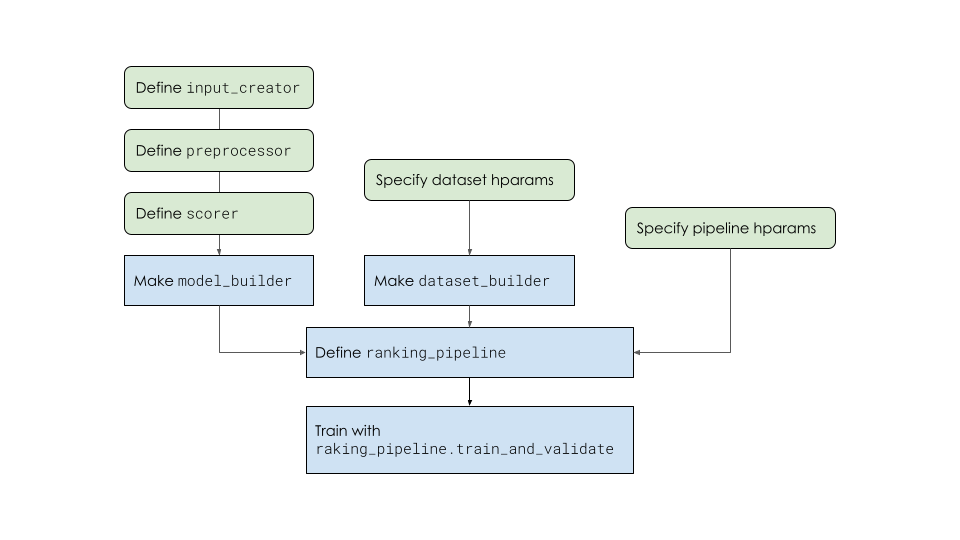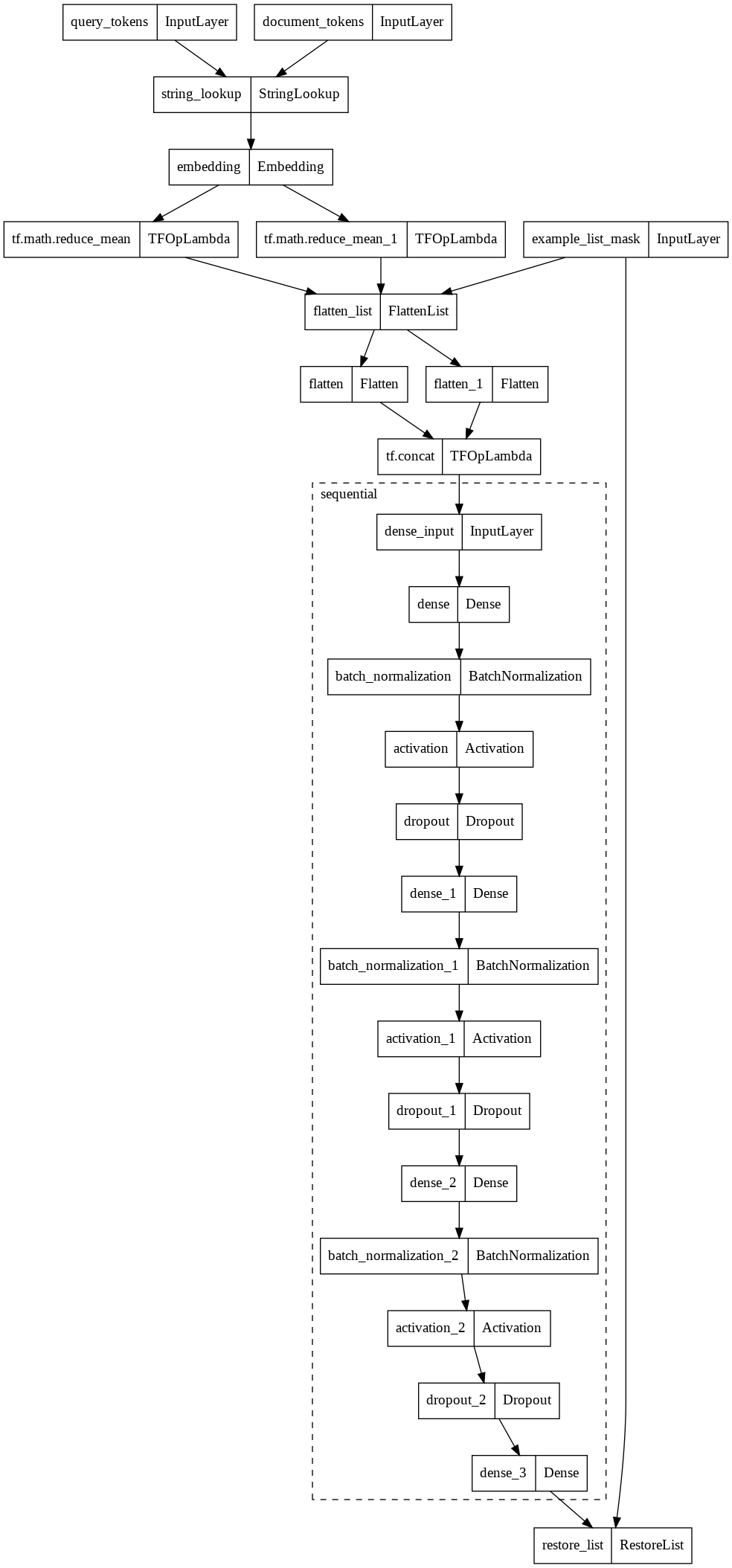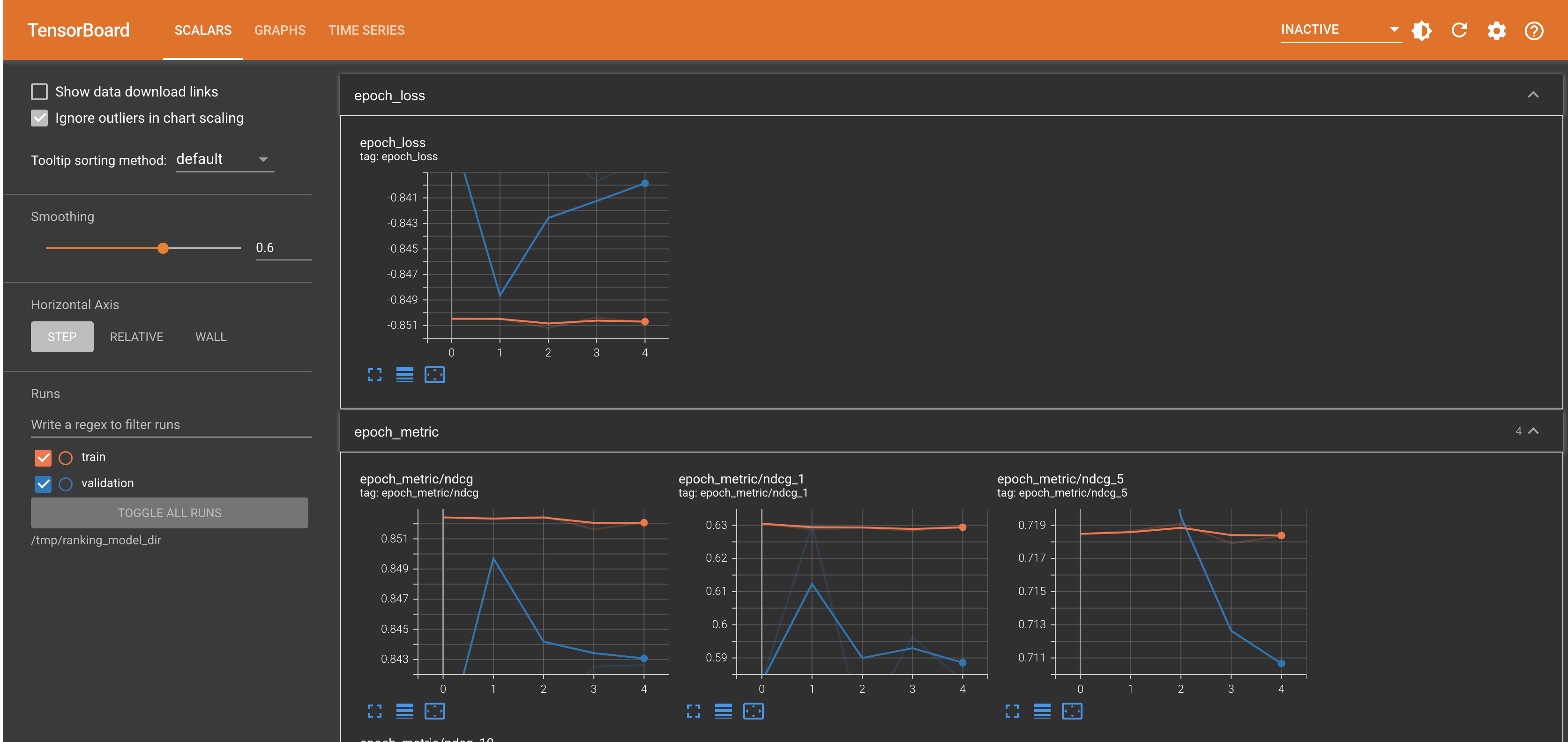# TensorFlow Ranking Keras pipeline for distributed training

TensorFlow Ranking can handle heterogeneous dense and sparse features, and scales up to millions of data points. However, building and deploying a learning to rank model to operate at scale creates additional challenges beyond simply designing a model. The Ranking library provides workflow utility classes for building distributed training for large-scale ranking applications. For more information about these features, see the TensorFlow Ranking Overview.

This tutorial shows you how to build a ranking model that enables a distributed processing strategy by using the Ranking library's support for a pipeline processing architecture.

## ANTIQUE dataset

In this tutorial, you will build a ranking model for ANTIQUE, a question-answering dataset. Given a query, and a list of answers, the objective is to rank the answers with optimal rank related metrics, such as NDCG. For more details about ranking metrics, review evaluation measures offline metrics.

ANTIQUE is a publicly available dataset for open-domain non-factoid question answering, collected from Yahoo! answers. Each question has a list of answers, whose relevance are graded on a scale of 0-4, 0 for irrelevant and 4 for fully relevant. The list size can vary depending on the query, so we use a fixed "list size" of 50, where the list is either truncated or padded with default values. The dataset is split into 2206 queries for training and 200 queries for testing. For more details, please read the technical paper on arXiv.

## Setup

````pip install -q tensorflow-ranking tensorflow-serving-api`
`pip install -U "tensorflow-text==2.11.*"`
```

Import TensorFlow Ranking library and useful libraries through the notebook.

``````import pathlib

import tensorflow as tf
import tensorflow_ranking as tfr
import tensorflow_text as tf_text
from tensorflow_serving.apis import input_pb2
``````

## Data preparation

````wget -O "/tmp/train.tfrecords" "http://ciir.cs.umass.edu/downloads/Antique/tf-ranking/ELWC/train.tfrecords"`
`wget -O "/tmp/test.tfrecords" "http://ciir.cs.umass.edu/downloads/Antique/tf-ranking//ELWC/test.tfrecords"`
`wget -O "/tmp/vocab.txt" "http://ciir.cs.umass.edu/downloads/Antique/tf-ranking/vocab.txt"`
```

Here, the dataset is saved in a ranking-specific ExampleListWithContext (ELWC) format. Detailed in the next section, shows how to generate and store data in the ELWC format.

### ELWC Data Formats for Ranking

The data for a single question consists of a list of `query_tokens` representing the question (the "context"), and a list of answers (the "examples"). Each answer is represented as a list of `document_tokens` and a `relevance` score. The following code shows a simplified representation of a question's data:

``````example_list_with_context = {
"context": {
"query_tokens": ["this", "is", "a", "question"]
},
"examples": [
{
"document_tokens": ["this", "is", "a", "relevant", "answer"],
"relevance": 
},
{
"document_tokens": ["irrelevant", "data"],
"relevance": 
}
]
}
``````

The data files, downloaded in the previous section, contain a serialized protobuffer representation of this sort of data. These protobuffers are quite long when viewed as text, but encode the same data.

``````CONTEXT = text_format.Parse(
"""
features {
feature {
key: "query_tokens"
value { bytes_list { value: ["this", "is", "a", "question"] } }
}
}""", tf.train.Example())
``````
``````EXAMPLES = [
text_format.Parse(
"""
features {
feature {
key: "document_tokens"
value { bytes_list { value: ["this", "is", "a", "relevant", "answer"] } }
}
feature {
key: "relevance"
value { int64_list { value: 4 } }
}
}""", tf.train.Example()),
text_format.Parse(
"""
features {
feature {
key: "document_tokens"
value { bytes_list { value: ["irrelevant", "data"] } }
}
feature {
key: "relevance"
value { int64_list { value: 0 } }
}
}""", tf.train.Example()),
]
``````
``````ELWC = input_pb2.ExampleListWithContext()
ELWC.context.CopyFrom(CONTEXT)
for example in EXAMPLES:
example_features.CopyFrom(example)

print(ELWC)
``````
```examples {
features {
feature {
key: "document_tokens"
value {
bytes_list {
value: "this"
value: "is"
value: "a"
value: "relevant"
}
}
}
feature {
key: "relevance"
value {
int64_list {
value: 4
}
}
}
}
}
examples {
features {
feature {
key: "document_tokens"
value {
bytes_list {
value: "irrelevant"
value: "data"
}
}
}
feature {
key: "relevance"
value {
int64_list {
value: 0
}
}
}
}
}
context {
features {
feature {
key: "query_tokens"
value {
bytes_list {
value: "this"
value: "is"
value: "a"
value: "question"
}
}
}
}
}
```

While the text format is verbose, protos can be efficiently serialized to a byte string (and parsed back into a proto)

``````serialized_elwc = ELWC.SerializeToString()
print(serialized_elwc)
``````
```b"\nL\nJ\n4\n\x0fdocument_tokens\x12!\n\x1f\n\x04this\n\x02is\n\x01a\n\x08relevant\n\x06answer\n\x12\n\trelevance\x12\x05\x1a\x03\n\x01\x04\n?\n=\n\x12\n\trelevance\x12\x05\x1a\x03\n\x01\x00\n'\n\x0fdocument_tokens\x12\x14\n\x12\n\nirrelevant\n\x04data\x12-\n+\n)\n\x0cquery_tokens\x12\x19\n\x17\n\x04this\n\x02is\n\x01a\n\x08question"
```

The following parser configuration parses the binary representation into a dictionary of tensors:

``````def parse_elwc(elwc):
return tfr.data.parse_from_example_list(
[elwc],
list_size=2,
context_feature_spec={"query_tokens": tf.io.RaggedFeature(dtype=tf.string)},
example_feature_spec={
"document_tokens":
tf.io.RaggedFeature(dtype=tf.string),
"relevance":
tf.io.FixedLenFeature(shape=[], dtype=tf.int64, default_value=0)
},
size_feature_name="_list_size_",

parse_elwc(serialized_elwc)
``````
```{'_list_size_': <tf.Tensor: shape=(1,), dtype=int32, numpy=array(, dtype=int32)>,
'_mask_': <tf.Tensor: shape=(1, 2), dtype=bool, numpy=array([[ True,  True]])>,
'document_tokens': <tf.RaggedTensor [[[b'this', b'is', b'a', b'relevant', b'answer'], [b'irrelevant', b'data']]]>,
'query_tokens': <tf.RaggedTensor [[b'this', b'is', b'a', b'question']]>,
'relevance': <tf.Tensor: shape=(1, 2), dtype=int64, numpy=array([[4, 0]])>}
```

Note with ELWC, you could also generate `size` and/or `mask` features to indicate the valid size and/or to mask out the valid entries in the list as long as `size_feature_name` and/or `mask_feature_name` are defined.

The above parser is defined in `tfr.data` and wrapped in our predefined dataset builder `tfr.keras.pipeline.BaseDatasetBuilder`.

## Overview of the ranking pipeline

Follow the steps depicted in the figure below to train a ranking model with ranking pipeline. In particular, this example uses the `tfr.keras.model.FeatureSpecInputCreator` and `tfr.keras.pipeline.BaseDatasetBuilder` defined specific for the datasets with `feature_spec`.## Create a model builder

Instead of directly building a `tf.keras.Model` object, create a model_builder, which is called in the ranking pipeline to build the `tf.keras.Model`, as all training parameters must be defined under the `strategy.scope` (called in `train_and_validate` function in ranking pipeline) in order to train with distributed strategies.

This framework uses the keras functional api to build models, where inputs (`tf.keras.Input`), preprocessors (`tf.keras.layers.experimental.preprocessing`), and scorer (`tf.keras.Sequential`) are required to define the model.

### Specify Features

Feature Specification are TensorFlow abstractions that are used to capture rich information about each feature.

Create feature specifications for context features, example features, and labels, consistent with the input formats for ranking, such as ELWC format.

The `default_value` of `label_spec` feature is set to -1 to take care of the padding items to be masked out.

``````context_feature_spec = {
"query_tokens": tf.io.RaggedFeature(dtype=tf.string),
}
example_feature_spec = {
"document_tokens":
tf.io.RaggedFeature(dtype=tf.string),
}
label_spec = (
"relevance",
tf.io.FixedLenFeature(shape=(1,), dtype=tf.int64, default_value=-1)
)
``````

### Define `input_creator`

`input_creator` create dictionaries of context and example `tf.keras.Input`s for input features defined in `context_feature_spec` and `example_feature_spec`.

``````input_creator = tfr.keras.model.FeatureSpecInputCreator(
context_feature_spec, example_feature_spec)
``````

Callling the `input_creator` returns the dictionaries of Keras-Tensors, that are used as the inputs when building the model:

``````input_creator()
``````
```({'query_tokens': <KerasTensor: type_spec=RaggedTensorSpec(TensorShape([None, None]), tf.string, 1, tf.int64) (created by layer 'query_tokens')>},
{'document_tokens': <KerasTensor: type_spec=RaggedTensorSpec(TensorShape([None, None, None]), tf.string, 2, tf.int64) (created by layer 'document_tokens')>})
```

### Define `preprocessor`

In the `preprocessor`, the input tokens are converted to a one-hot vector through the String Lookup preprocessing layer and then embeded as an embedding vector through the Embedding preprocessing layer. Finally, compute an embedding vector for the full sentence by the average of token embeddings.

``````class LookUpTablePreprocessor(tfr.keras.model.Preprocessor):

def __init__(self, vocab_file, vocab_size, embedding_dim):
self._vocab_file = vocab_file
self._vocab_size = vocab_size
self._embedding_dim = embedding_dim

lookup = tf.keras.layers.StringLookup(
max_tokens=self._vocab_size,
vocabulary=self._vocab_file,
embedding = tf.keras.layers.Embedding(
input_dim=self._vocab_size,
output_dim=self._embedding_dim,
embeddings_initializer=None,
embeddings_constraint=None)
# StringLookup and Embedding are shared over context and example features.
context_features = {
key: tf.reduce_mean(embedding(lookup(value)), axis=-2)
for key, value in context_inputs.items()
}
example_features = {
key: tf.reduce_mean(embedding(lookup(value)), axis=-2)
for key, value in example_inputs.items()
}
return context_features, example_features
``````
``````_VOCAB_FILE = '/tmp/vocab.txt'
``````
``````preprocessor = LookUpTablePreprocessor(_VOCAB_FILE, _VOCAB_SIZE, 20)
``````

Note that the vocabulary uses the same tokenizer that BERT does. You could also use BertTokenizer to tokenize the raw sentences.

``````tokenizer = tf_text.BertTokenizer(_VOCAB_FILE)
example_tokens = tokenizer.tokenize("Hello TensorFlow!".lower())

print(example_tokens)
print(tokenizer.detokenize(example_tokens))
``````
```<tf.RaggedTensor [[, [23435, 12314], ]]>
<tf.RaggedTensor [[[b'hello'], [b'tensorflow'], [b'!']]]>
```

### Define `scorer`

This example uses a Deep Neural Network (DNN) univariate scorer, predefined in TensorFlow Ranking.

``````scorer = tfr.keras.model.DNNScorer(
hidden_layer_dims=[64, 32, 16],
output_units=1,
activation=tf.nn.relu,
use_batch_norm=True)
``````

### Make `model_builder`

In addition to `input_creator`, `preprocessor`, and `scorer`, specify the mask feature name to take the mask feature generated in datasets.

``````model_builder = tfr.keras.model.ModelBuilder(
input_creator=input_creator,
preprocessor=preprocessor,
scorer=scorer,
name="antique_model",
)
``````

Check the model architecture,

``````model = model_builder.build()
tf.keras.utils.plot_model(model, expand_nested=True)
``````## Create a dataset builder

A `dataset_builder` is designed to create datasets for training and validation and to define signatures for exporting trained model as `tf.function`.

### Specify data hyperparameters

Define the hyperparameters to be used to build datasets in `dataset_builder` by creating a `dataset_hparams` object.

Load training dataset at `/tmp/train.tfrecords` with `tf.data.TFRecordDataset` reader. In each batch, each feature tensor has a shape `(batch_size, list_size, feature_sizes)` with `batch_size` equal to 32 and `list_size` equal to 50. Validate with the test data at `/tmp/test.tfrecords` at the same `batch_size` and `list_size`.

``````dataset_hparams = tfr.keras.pipeline.DatasetHparams(
train_input_pattern="/tmp/train.tfrecords",
valid_input_pattern="/tmp/test.tfrecords",
train_batch_size=32,
valid_batch_size=32,
list_size=50,
``````

### Make `dataset_builder`

TensorFlow Ranking provides a pre-defined `SimpleDatasetBuilder` to generate datasets from ELWC using `feature_spec`s. As a mask feature is used to determine valid examples in each padded list, must specify the `mask_feature_name` consistent with the `mask_feature_name` used in `model_builder`.

``````dataset_builder = tfr.keras.pipeline.SimpleDatasetBuilder(
context_feature_spec,
example_feature_spec,
label_spec=label_spec,
hparams=dataset_hparams)
``````
``````ds_train = dataset_builder.build_train_dataset()
ds_train.element_spec
``````
```({'document_tokens': RaggedTensorSpec(TensorShape([None, 50, None]), tf.string, 2, tf.int32),
'query_tokens': RaggedTensorSpec(TensorShape([32, None]), tf.string, 1, tf.int32)},
TensorSpec(shape=(32, 50), dtype=tf.float32, name=None))
```

## Create a ranking pipeline

A `ranking_pipeline` is an optimized ranking model training package that implement distributed training, export model as `tf.function`, and integrate useful callbacks including tensorboard and restoring upon failures.

### Specify pipeline hyperparameters

Specify the hyperparameters to be used to run the pipeline in `ranking_pipeline` by creating a `pipeline_hparams` object.

Train the model with `approx_ndcg_loss` at learning rate equal to 0.05 for 5 epoch with 1000 steps in each epoch using `MirroredStrategy`. Evaluate the model on the validation dataset for 100 steps after each epoch. Save the trained model under `/tmp/ranking_model_dir`.

``````pipeline_hparams = tfr.keras.pipeline.PipelineHparams(
model_dir="/tmp/ranking_model_dir",
num_epochs=5,
steps_per_epoch=1000,
validation_steps=100,
learning_rate=0.05,
loss="approx_ndcg_loss",
strategy="MirroredStrategy")
``````

### Define `ranking_pipeline`

TensorFlow Ranking provides a pre-defined `SimplePipeline` to support model training with distributed strategies.

``````ranking_pipeline = tfr.keras.pipeline.SimplePipeline(
model_builder,
dataset_builder=dataset_builder,
hparams=pipeline_hparams)
``````
```WARNING:tensorflow:There are non-GPU devices in `tf.distribute.Strategy`, not using nccl allreduce.
WARNING:tensorflow:There are non-GPU devices in `tf.distribute.Strategy`, not using nccl allreduce.
```

## Train and evaluate the model

The `train_and_validate` function evaluates the trained model on the validation dataset after every epoch.

``````ranking_pipeline.train_and_validate(verbose=1)
``````
```Epoch 1/5
"shape. This may consume a large amount of memory." % value)
"shape. This may consume a large amount of memory." % value)
1000/1000 [==============================] - 121s 121ms/step - loss: -0.8845 - metric/ndcg_1: 0.7122 - metric/ndcg_5: 0.7813 - metric/ndcg_10: 0.8413 - metric/ndcg: 0.8856 - val_loss: -0.8672 - val_metric/ndcg_1: 0.6557 - val_metric/ndcg_5: 0.7689 - val_metric/ndcg_10: 0.8243 - val_metric/ndcg: 0.8678
Epoch 2/5
1000/1000 [==============================] - 88s 88ms/step - loss: -0.8957 - metric/ndcg_1: 0.7428 - metric/ndcg_5: 0.8005 - metric/ndcg_10: 0.8551 - metric/ndcg: 0.8959 - val_loss: -0.8731 - val_metric/ndcg_1: 0.6614 - val_metric/ndcg_5: 0.7812 - val_metric/ndcg_10: 0.8348 - val_metric/ndcg: 0.8733
Epoch 3/5
1000/1000 [==============================] - 50s 50ms/step - loss: -0.8955 - metric/ndcg_1: 0.7422 - metric/ndcg_5: 0.7991 - metric/ndcg_10: 0.8545 - metric/ndcg: 0.8957 - val_loss: -0.8695 - val_metric/ndcg_1: 0.6414 - val_metric/ndcg_5: 0.7759 - val_metric/ndcg_10: 0.8315 - val_metric/ndcg: 0.8699
Epoch 4/5
1000/1000 [==============================] - 53s 53ms/step - loss: -0.9009 - metric/ndcg_1: 0.7563 - metric/ndcg_5: 0.8094 - metric/ndcg_10: 0.8620 - metric/ndcg: 0.9011 - val_loss: -0.8624 - val_metric/ndcg_1: 0.6179 - val_metric/ndcg_5: 0.7627 - val_metric/ndcg_10: 0.8253 - val_metric/ndcg: 0.8626
Epoch 5/5
1000/1000 [==============================] - 52s 52ms/step - loss: -0.9042 - metric/ndcg_1: 0.7646 - metric/ndcg_5: 0.8152 - metric/ndcg_10: 0.8662 - metric/ndcg: 0.9044 - val_loss: -0.8733 - val_metric/ndcg_1: 0.6579 - val_metric/ndcg_5: 0.7741 - val_metric/ndcg_10: 0.8362 - val_metric/ndcg: 0.8741
INFO:tensorflow:Assets written to: /tmp/ranking_model_dir/export/latest_model/assets
INFO:tensorflow:Assets written to: /tmp/ranking_model_dir/export/latest_model/assets
```

### Launch TensorBoard

``````%load_ext tensorboard
%tensorboard --logdir="/tmp/ranking_model_dir" --port 12345
``````### Generate predictions and evaluate

Get the test data.

``````ds_test = dataset_builder.build_valid_dataset()

# Get input features from the first batch of the test data
for x, y in ds_test.take(1):
break
``````

Load the saved model and run a prediction.

``````loaded_model = tf.keras.models.load_model("/tmp/ranking_model_dir/export/latest_model")
``````
``````# Predict ranking scores
min_score = tf.reduce_min(scores)
scores = tf.where(tf.greater_equal(y, 0.), scores, min_score - 1e-5)

# Sort the answers by scores
scores,
[tf.strings.reduce_join(x['document_tokens'], -1, separator=' ')])
``````

Check the top 5 answers for question number 4.

``````question = tf.strings.reduce_join(
x['query_tokens'][4, :], -1, separator=' ').numpy()

print(
f'Q: {question.decode()}\n' +
'\n'.join([f'A{i+1}: {ans.decode()}' for i, ans in enumerate(top_answers)]))
``````
```Q: why do people ask questions they know ?
A1: because it re ##as ##ures them that they were right in the first place .
A2: people like to that be ##cao ##use they want to be recognise that they are the one knows the answer and the questions int ##he first place .
A3: to rev ##ali ##date their knowledge and perhaps they choose answers that are mostly with their side simply because they are being subjective . . . .
A4: so they can weasel out the judge mental and super ##ci ##lio ##us know all cr ##aa ##p like yourself . . . don ##t judge others , what gives you the right ? . . how do you know what others know . ? . . by asking this question you are putting yourself in the same league as the others you want ot condemn . . face it you already know what your shallow , self absorbed answer is . . . get a reality check pill ##ock , . . . and if you want to go gr ##iz ##z ##ling to the yahoo policeman bring it on . . it will only reinforce my answer and the pathetic ##iness of your q ##est ##ion . . . the only thing you could do that would be even more pathetic is give me the top answer award . . . then you would suck beyond all measure
A5: human nature i guess . i have noticed that too . maybe it is just for re ##ass ##urance or approval .
```
[]
[]